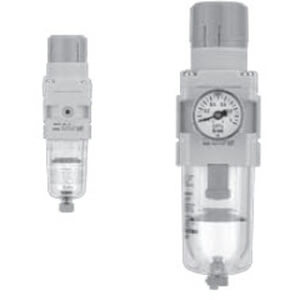# AW20(*)-B to AW60(*)-B, Filter Regulator & Filter Regulator w/Backflow FunctionSMC'S series AW-B is a combination filter/regulator, minimizing space and piping by integrating two units into one. The AW-B series has a maximum set pressure of 125 psi and offers embedded gauge and pressure switch options not found in the AW-A while maintaining panel mounting interchangeability with the previous AW models. Bowls on the size 30 and up are covered with a transparent bowl guard, completely protecting them from the environment and making the interior contents visible from 360 degrees. This series is part of the family of SMC modular air preparation units and can be combined with other similar sized product. The new AW-B is available in four body sizes with piping from 1/8" to 1" in Rc, NPT and G thread types.

Configuration OptionsCopy Configuration Number

## Product Options

Select Product Options
 Select values from the drop down lists or make appropriate selections to configure the part number. Body Size -- Pick an Option -- 20 30 40 60Backflow Function -- Pick an Option -- None K (w/Backflow Function)Thread -- Pick an Option -- Rc N (NPT) F (G)Port -- Pick an Option -- 01 (1/8) 02 (1/4) 03 (3/8) 04 (1/2) 06 (3/4) 10 (1)Option -- Pick an Option -- Standard B (w/Bracket) H (w/Set Nut, for Panel Mount) C [Float Type Auto Drain (N.C.)] D [Float Type Auto Drain (N.O.)] E [Square embedded type pressure gauge (with limit indicator)] E1 (Output: NPN output / Electrical entry: Wiring bottom entry) E2 (Output: NPN output / Electrical entry: Wiring top entry) E3 (Output: PNP output / Electrical entry: Wiring bottom entry) E4 (Output: PNP output / Electrical entry: Wiring top entry) G (Round Pressure Gauge w/Limit Indicator) M [Round type pressure gauge (with color zone)] BC (B + C) BCE (B + C + E) BCE1 (B + C + E1) BCE2 (B + C + E2) BCE3 (B + C + E3) BCE4 (B + C + E4) BCG (B + C + G) BD (B + D) BDE (B + D + E) BDE1 (B + D + E1) BDE2 (B + D + E2) BDE3 (B + D + E3) BDE4 (B + D + E4) BDG (B + D + G) BDH (B + D + H) BDM (B + D + M) BE (B + E) BE1 (B + E1) BE2 (B + E2) BE3 (B + E3) BE4 (B + E4) BG (B + G) BGH (B + G + H) BGM (B + G + M) BM (B + M) CE (C + E) CE1 (C + E1) CE1H (C + E1 + H) CE2 (C + E2) CE2H (C + E2 + H) CE3 (C + E3) CE3H (C + E3 + H) CE4 (C + E4) CE4H (C + E4 + H) CEH (C + E + H) CG (C + G) CGH (C + G + H) CH (C + H) CHM (C + H + M) CM (C + M) DE (D + E) DE1 ( D + E1) DE2 (D + E2) DE3 (D + E3) DE4 (D + E4) DEH (D + E + H) DG (D + G) DH (D + H) DM (D + M) E1H (E1 + H) E2H (E2 + H) E3H (E3 + H) E4H (E4 + H) EH (E + H) EHM (E + H + M) GH (G + H) GHM (G + H + M) HM (H + M)Semi-Standard 1 -- Pick an Option -- Standard 1 (Set Pressure: 0.02 to 0.2 MPa) 2 (Metal Bowl) C (Bowl Guard) 6 (Nylon Bowl) 6C [Bowl Guard (Nylon Bowl)] 8 (Metal Bowl w/Level Gauge) J (Drain Guide 1/8: Size 20; Drain Guide 1/4: Sizes 30, 40, 60) 12 (1 + 2) 12J (1 + 2 + J) 16 (1 + 6) 16C (1 + 6 + C) 16CJ (1 + 6 + C + J) 16J (1 + 6 + J) 18 (1 + 8) 18J (1 + 8 + J) 1C (1 + C) 1CJ (1 + C + J) 1J (1 + J) 2J (2 + J) CJ (C + J) 6CJ (6C + J) 6J (6 + J) 8J (8 + J)Semi-Standard 2 -- Pick an Option -- Standard Z (Name Plate, Caution Plate for Bowl, and Pressure Gauge: psi, °F) ZA (Digital Pressure Switch: With Unit Conversion Function) N (Exhaust Mechanism, Non-relieving) R (Flow Direction: Right->Left) W (Drain Cock w/Barb Fitting for ø6 x ø4 Nylon Tube) NR (N + R) NRW (N + R + W) NRWZ (N + R + W + Z) NRWZA (N + R + W + ZA) NRZ (N + R + Z) NRZA (N + R + ZA) NW (N + W) NWZ (N + W + Z) NWZA (N + W + ZA) NZ (N + Z) NZA (N + ZA) RZ (R + Z) RZA (R + ZA) RW (R + W) RWZ (R + W + Z) RWZA (R + W + ZA) WZ (W + Z) WZA (W + ZA)Options in: Gray are not compatible with other selected option value. Red conflict with another selected option value. Amber are not recommended.

The part number needs to be complete in order to view/download CAD Models.

Model does not exist, please email cad@smcusa.com with the complete part number, CAD file format and version required.